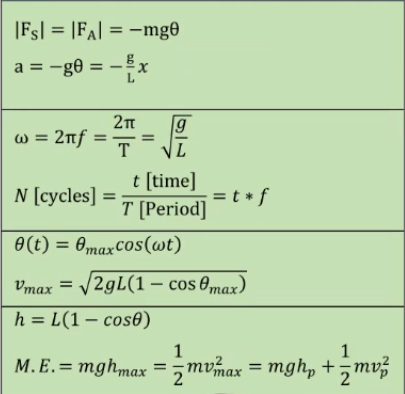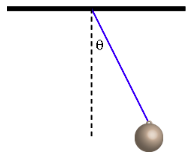# Problem: A simple pendulum with mass m = 2.3 kg and length L = 2.6 m hangs from the ceiling. It is pulled back to a small angle of θ = 9.1° from the vertical and released at t = 0. 1) What is the period of oscillation?2) What is the magnitude of the force on the pendulum bob perpendicular to the string at t = 0?3) What is the maximum speed of the pendulum? 4) What is the angular displacement at t = 3.53 s? (give the answer as a negative angle if the angle is to the left of the vertical) 5) What is the magnitude of the tangential acceleration as the pendulum passes through the equilibrium position? 6) What is the magnitude of the radial acceleration as the pendulum passes through the equilibrium position? 7) Which of the following would change the frequency of oscillation of this simple pendulum?A. increasing the mass B. decreasing the initial angular displacement C. increasing the length D. hanging the pendulum in an elevator accelerating downward

###### FREE Expert Solution

This problem is all about the harmonic motion of a simple pendulum.

We'll need various relations:93% (245 ratings)###### Problem Details

A simple pendulum with mass m = 2.3 kg and length L = 2.6 m hangs from the ceiling. It is pulled back to a small angle of θ = 9.1° from the vertical and released at t = 0.1) What is the period of oscillation?

2) What is the magnitude of the force on the pendulum bob perpendicular to the string at t = 0?

3) What is the maximum speed of the pendulum?

4) What is the angular displacement at t = 3.53 s? (give the answer as a negative angle if the angle is to the left of the vertical)

5) What is the magnitude of the tangential acceleration as the pendulum passes through the equilibrium position?

6) What is the magnitude of the radial acceleration as the pendulum passes through the equilibrium position?

7) Which of the following would change the frequency of oscillation of this simple pendulum?

A. increasing the mass

B. decreasing the initial angular displacement

C. increasing the length

D. hanging the pendulum in an elevator accelerating downward